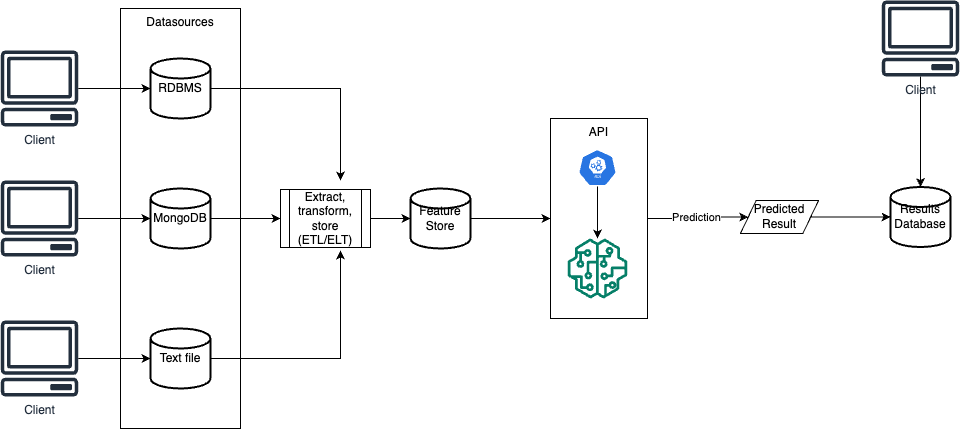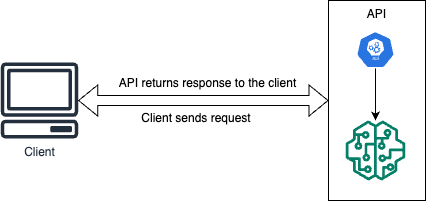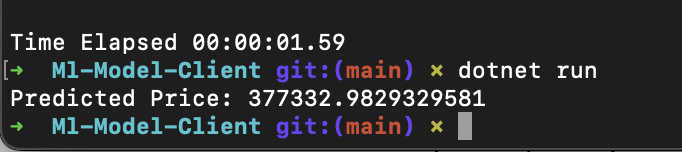#Photo by Kevin Ku on Unsplash

# Productionization of Machine Learning Models

### Introduction

Deploying machine learning models is essential for making them useful to the public or expected users. In this article, we will explore the best ways to deploy your models for production scenarios.

There are two common architectural approaches to deploying models:

1. Batch Mode

To predict outcomes, we store variables in a datastore during batch mode. The batch runs on a scheduled interval and retrieves variables from the feature store to make predictions. These predictions are saved to a data store for client applications to access by simply polling the data store for predicted values.2. Real-time Mode

Our model is integrated into an API, enabling real-time predictions. The API endpoint is called with the independent variables included in the payload, the API endpoint will provide the predicted result.Let's get started!

### Creating our model

First and foremost, we would create the model we will deploy. We will be building a simple linear regression to predict house prices in House Sales in King County, USA. The dataset is available here.

• import useful libraries

• ``````      import numpy as np
import pandas as pd
from sklearn.model_selection import train_test_split
from sklearn.linear_model import LinearRegression
import pickle
``````
• import our dataset

``````  # Load the Kings County House Sales dataset
``````
• building our model

``````
# Using 'sqft_living' as feature and 'price' as target
X = data["sqft_living"].values.reshape(-1, 1)
y = data["price"].values

# Split the data into training and testing sets
X_train, X_test, y_train, y_test = train_test_split(
X, y, test_size=0.2, random_state=42
)

# Create a linear regression model
model = LinearRegression()

# Fit the model to the training data
model.fit(X_train, y_train)
``````

### Saving our model

To seamlessly load and utilize our model, we must first serialize it. Software engineering defines serialization as saving an object from memory, enabling ease of access and use. We will save our model - currently only in the computer's memory - to the disk and then load it as needed. Our preferred method for serialization is through the use of the Pickle library.

• Install and import Pickle library.

``````  import pickle
``````
• Save our model.

``````  # Save the model using pickle
with open("kings_county_model.pkl", "wb") as model_file:
pickle.dump(model, model_file)
``````

### Exposing our model via APIs

The next step in the process is to expose our models via an API endpoint. We would be using Flask API here.

• Install and import Flask library.

``````  pip install Flask scikit-learn numpy
``````
• Create our endpoint and call our ML model.

``````  from flask import Flask, request, jsonify
import pickle
import numpy as np

with open('kings_county_model.pkl', 'rb') as model_file:

@app.route('/predict', methods=['POST'])
def predict():
data = request.get_json(force=True)
new_data = np.array(data['data']).reshape(-1, 1)
return jsonify(prediction.tolist())

if __name__ == '__main__':
app.run(debug=True)
``````

### Calling our model via a client

To consume our model, we'll create a client. For this purpose, we'll use a .NET C# client in this article, but feel free to use any programming language of your choice.

• Create a new C# console project. We will call ours `ML-Model-Client`

``````  dotnet new console -n 'ML-Model-Client'
``````

Call the Model's endpoint

Add the following lines of code to the `Program.cs file`.

``````  using System;
using System.Net.Http;
using System.Net.Http.Json;
using System.Text.Json;

class Program
{
{
// Define the API endpoint URL
var apiUrl = "http://127.0.0.1:5000/predict";

// Input data for prediction
var input = new { data = new[] { 1500 } }; // Example input data (sqft_living)

using HttpClient client = new HttpClient();
try
{
var response = await client.PostAsJsonAsync(apiUrl, input);

if (response.IsSuccessStatusCode)
{
Console.WriteLine(\$"Predicted Price: {string.Join(", ", predictedPrice)}");
}
else
{
Console.WriteLine("Request failed with status code: " + response.StatusCode);
}
}
catch (Exception ex)
{
Console.WriteLine(\$"Error: {ex.Message}");
}
}
}
``````

### Putting it All Together

To run the files, follow these steps:

1. Running `model_building.py`:

Open a terminal/command prompt and navigate to the directory where `model_building.py` is located. Then, run the script using the Python interpreter:

`````` python model_building.py
``````

This will build and export the linear regression model as `kings_county_model.pkl` .

2. Running the Flask App (`app.py`):

Open a new terminal/command prompt and navigate to the directory where `app.py` is located. Then run the Flask app using the Python interpreter:

`````` python app.py
``````

The Flask app will start, and the API will be accessible at `http://127.0.0.1:5000`.

3. Running the C# Client:

You'll need to compile and run the C# code using the dotnet CLI. Here are the steps:

• Navigate inside the `ML-Model-Client` folder

``````  dotnet build
``````
• Run the compiled C# client:

``````  dotnet run
``````

The C# client will send a POST request to the Flask API, receive the prediction response, and print the predicted price on the console. Here's the result of running mine:Remember that the steps above assume you have installed the necessary software tools, including Python, Flask, and .NET SDK. Additionally, ensure that you run the scripts in the correct directories and that the Flask app is running before testing the C# client.

### Conclusion

In this article, we have examined how to utilize our ML models in a production scenario. The code for this tutorial is available here.